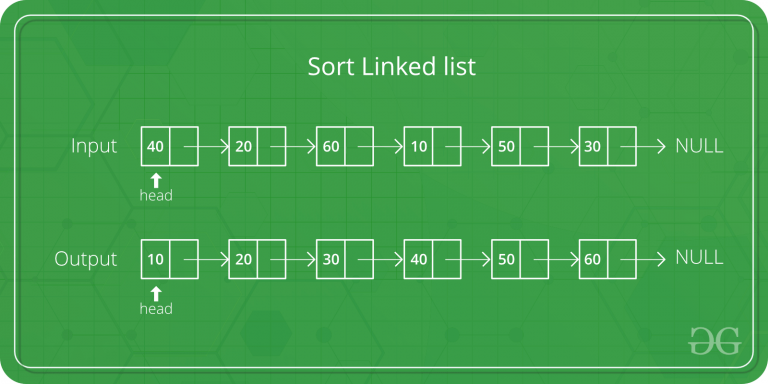GeeksforGeeks App
Open AppBrowser
Continue

# Java Program for Merge Sort for Linked Lists

Merge sort is often preferred for sorting a linked list. The slow random-access performance of a linked list makes some other algorithms (such as quicksort) perform poorly, and others (such as heapsort) completely impossible.Let head be the first node of the linked list to be sorted and headRef be the pointer to head. Note that we need a reference to head in MergeSort() as the below implementation changes next links to sort the linked lists (not data at the nodes), so head node has to be changed if the data at original head is not the smallest value in linked list.

```MergeSort(headRef)
1) If head is NULL or there is only one element in the Linked List
then return.
2) Else divide the linked list into two halves.
FrontBackSplit(head, &a, &b); /* a and b are two halves */
3) Sort the two halves a and b.
MergeSort(a);
MergeSort(b);
4) Merge the sorted a and b (using SortedMerge() discussed here)
```

## Java

 `// Java program to illustrate merge sorted``// of linkedList`` ` `public` `class` `linkedList {``    ``node head = ``null``;``    ``// node a, b;``    ``static` `class` `node {``        ``int` `val;``        ``node next;`` ` `        ``public` `node(``int` `val)``        ``{``            ``this``.val = val;``        ``}``    ``}`` ` `    ``node sortedMerge(node a, node b)``    ``{``        ``node result = ``null``;``        ``/* Base cases */``        ``if` `(a == ``null``)``            ``return` `b;``        ``if` `(b == ``null``)``            ``return` `a;`` ` `        ``/* Pick either a or b, and recur */``        ``if` `(a.val <= b.val) {``            ``result = a;``            ``result.next = sortedMerge(a.next, b);``        ``}``        ``else` `{``            ``result = b;``            ``result.next = sortedMerge(a, b.next);``        ``}``        ``return` `result;``    ``}`` ` `    ``node mergeSort(node h)``    ``{``        ``// Base case : if head is null``        ``if` `(h == ``null` `|| h.next == ``null``) {``            ``return` `h;``        ``}`` ` `        ``// get the middle of the list``        ``node middle = getMiddle(h);``        ``node nextofmiddle = middle.next;`` ` `        ``// set the next of middle node to null``        ``middle.next = ``null``;`` ` `        ``// Apply mergeSort on left list``        ``node left = mergeSort(h);`` ` `        ``// Apply mergeSort on right list``        ``node right = mergeSort(nextofmiddle);`` ` `        ``// Merge the left and right lists``        ``node sortedlist = sortedMerge(left, right);``        ``return` `sortedlist;``    ``}`` ` `    ``// Utility function to get the middle of the linked list``    ``node getMiddle(node h)``    ``{``        ``// Base case``        ``if` `(h == ``null``)``            ``return` `h;``        ``node fastptr = h.next;``        ``node slowptr = h;`` ` `        ``// Move fastptr by two and slow ptr by one``        ``// Finally slowptr will point to middle node``        ``while` `(fastptr != ``null``) {``            ``fastptr = fastptr.next;``            ``if` `(fastptr != ``null``) {``                ``slowptr = slowptr.next;``                ``fastptr = fastptr.next;``            ``}``        ``}``        ``return` `slowptr;``    ``}`` ` `    ``void` `push(``int` `new_data)``    ``{``        ``/* allocate node */``        ``node new_node = ``new` `node(new_data);`` ` `        ``/* link the old list off the new node */``        ``new_node.next = head;`` ` `        ``/* move the head to point to the new node */``        ``head = new_node;``    ``}`` ` `    ``// Utility function to print the linked list``    ``void` `printList(node headref)``    ``{``        ``while` `(headref != ``null``) {``            ``System.out.print(headref.val + ``" "``);``            ``headref = headref.next;``        ``}``    ``}`` ` `    ``public` `static` `void` `main(String[] args)``    ``{`` ` `        ``linkedList li = ``new` `linkedList();``        ``/*``         ``* Let us create a unsorted linked lists to test the functions Created``         ``* lists shall be a: 2->3->20->5->10->15``         ``*/``        ``li.push(``15``);``        ``li.push(``10``);``        ``li.push(``5``);``        ``li.push(``20``);``        ``li.push(``3``);``        ``li.push(``2``);``        ``System.out.println(``"Linked List without sorting is :"``);``        ``li.printList(li.head);`` ` `        ``// Apply merge Sort``        ``li.head = li.mergeSort(li.head);``        ``System.out.print(``"\n Sorted Linked List is: \n"``);``        ``li.printList(li.head);``    ``}``}`` ` `// This code is contributed by Rishabh Mahrsee`

Output:

```Linked List without sorting is :
2 3 20 5 10 15
2 3 5 10 15 20
```

Time Complexity: O(n*log n)

Space Complexity: O(n*log n)

Approach 2: This approach is simpler and uses log n space.

mergeSort():

1. If the size of the linked list is 1 then return the head
2. Find mid using The Tortoise and The Hare Approach
3. Store the next of mid in head2 i.e. the right sub-linked list.
4. Now Make the next midpoint null.
5. Recursively call mergeSort() on both left and right sub-linked list and store the new head of the left and right linked list.
6. Call merge() given the arguments new heads of left and right sub-linked lists and store the final head returned after merging.

1. Take a pointer say merged to store the merged list in it and store a dummy node in it.
2. Take a pointer temp and assign merge to it.
3. If the data of head1 is less than the data of head2, then, store head1 in next of temp & move head1 to the next of head1.
5. Move temp to the next of temp.
6. Repeat steps 3, 4 & 5 until head1 is not equal to null and head2 is not equal to null.
7. Now add any remaining nodes of the first or the second linked list to the merged linked list.
8. Return the next of merged(that will ignore the dummy and return the head of the final merged linked list)

## Java

 `// Java program for the above approach``import` `java.io.*;``import` `java.lang.*;``import` `java.util.*;`` ` `// Node Class``class` `Node {``    ``int` `data;``    ``Node next;``    ``Node(``int` `key)``    ``{``        ``this``.data = key;``        ``next = ``null``;``    ``}``}`` ` `class` `GFG {``   ` `    ``// Function to merge sort``    ``static` `Node mergeSort(Node head)``    ``{``        ``if` `(head.next == ``null``)``            ``return` `head;`` ` `        ``Node mid = findMid(head);``        ``Node head2 = mid.next;``        ``mid.next = ``null``;``        ``Node newHead1 = mergeSort(head);``        ``Node newHead2 = mergeSort(head2);``        ``Node finalHead = merge(newHead1, newHead2);`` ` `        ``return` `finalHead;``    ``}`` ` `    ``// Function to merge two linked lists``    ``static` `Node merge(Node head1, Node head2)``    ``{``        ``Node merged = ``new` `Node(-``1``);``        ``Node temp = merged;``       ` `        ``// While head1 is not null and head2``        ``// is not null``        ``while` `(head1 != ``null` `&& head2 != ``null``) {``            ``if` `(head1.data < head2.data) {``                ``temp.next = head1;``                ``head1 = head1.next;``            ``}``            ``else` `{``                ``temp.next = head2;``                ``head2 = head2.next;``            ``}``            ``temp = temp.next;``        ``}``       ` `        ``// While head1 is not null``        ``while` `(head1 != ``null``) {``            ``temp.next = head1;``            ``head1 = head1.next;``            ``temp = temp.next;``        ``}``       ` `        ``// While head2 is not null``        ``while` `(head2 != ``null``) {``            ``temp.next = head2;``            ``head2 = head2.next;``            ``temp = temp.next;``        ``}``        ``return` `merged.next;``    ``}`` ` `    ``// Find mid using The Tortoise and The Hare approach``    ``static` `Node findMid(Node head)``    ``{``        ``Node slow = head, fast = head.next;``        ``while` `(fast != ``null` `&& fast.next != ``null``) {``            ``slow = slow.next;``            ``fast = fast.next.next;``        ``}``        ``return` `slow;``    ``}`` ` `    ``// Function to print list``    ``static` `void` `printList(Node head)``    ``{``        ``while` `(head != ``null``) {``            ``System.out.print(head.data + ``" "``);``            ``head = head.next;``        ``}``    ``}`` ` `    ``// Driver Code``    ``public` `static` `void` `main(String[] args)``    ``{``        ``Node head = ``new` `Node(``7``);``        ``Node temp = head;``        ``temp.next = ``new` `Node(``10``);``        ``temp = temp.next;``        ``temp.next = ``new` `Node(``5``);``        ``temp = temp.next;``        ``temp.next = ``new` `Node(``20``);``        ``temp = temp.next;``        ``temp.next = ``new` `Node(``3``);``        ``temp = temp.next;``        ``temp.next = ``new` `Node(``2``);``        ``temp = temp.next;`` ` `        ``// Apply merge Sort``        ``head = mergeSort(head);``        ``System.out.print(``"\nSorted Linked List is: \n"``);``        ``printList(head);``    ``}``}`

Output:

```Sorted Linked List is:
2 3 5 7 10 20 ```

Time Complexity: O(n*log n)

Space Complexity: O(log n)

Please refer complete article on Merge Sort for Linked Lists for more details!

My Personal Notes arrow_drop_up